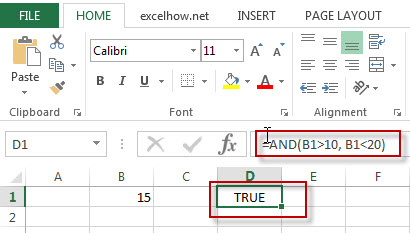# Excel And Function

This post will guide you how to use Excel AND function with syntax and examples in Microsoft excel.

### Description

The Excel AND function returns TRUE if all of arguments are TRUE, and it returns FALSE if any of arguments are FALSE.

The AND function is a build-in function in Microsoft Excel and it is categorized as a Logical Function.

The AND function is available in Excel 2016, Excel 2013, Excel 2010, Excel 2007, Excel 2003, Excel XP, Excel 2000, Excel 2011 for Mac.

### Syntax

The syntax of the AND function is as below:

= AND (condition1,[condition2],…)

Where the AND function arguments are:
Condition1 -This is a required argument. The first arguments to test if it is true.
Condition2 – Optional

### Example

The below examples will show you how to use Excel AND Function to test whether all of conditions are TRUE.

#1 To Test if B1 is greater than 10 AND less than 20, if so, returns TRUE value, just using the following excel formula: =AND(B1>10, B1<20)### More Excel AND Function Examples

• Conditional Formatting date with red Amber or Green
How to highlight the date with red if the cell dates is past now(). How to highlight the date with amber if the cell date is past now but within the next 6 months from now(). How to highlight the date with green if the cell date is more than 6 months from now…
• Check If Multiple Cells are Equal In Excel
Assuming that you have a list of data in range A1:C1, and you want to compare if these cells are equal, if so, then return True, otherwise, return False. You need to create an Excel array formula based on the AND function and the EXACT function…